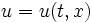# Min-increasing trajectory

This article defines a property that can be evaluated for a trajectory on the space of functions on a manifold

## Definition

Let$M$ be a manifold and$u = u(t,x)$ be a function$\R \times M \to \R$, where:

•$t$ denotes the time parameter, and varies in$\R$
•$x$ denotes the spatial parameter, and varies in$M$

In other words,$u$ is a trajectory (or path) in the space of all functions from$M$ to$\R$.

Then,$u$ is said to be min-increasing if the function:$t \mapsto \inf_{x \in M} u(t,x)$

is a monotone increasing function. (the function defined above is called the timewise-min function for$u$).

The corresponding notion is of a max-decreasing trajectory -- viz a trajectory where the maximum (or supremum) keeps decreasing.